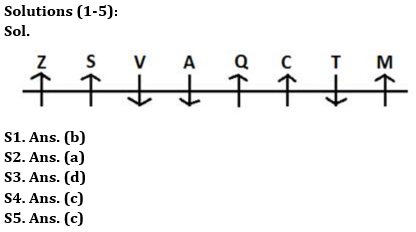Latest Banking jobs   »

# Reasoning Quiz For RBI Grade B Phase 1 2023-26th May

Directions (1-5): Study the following information carefully and answer the given questions.
Eight people sit in a row and some of them face towards north direction and some of them face towards south direction. Not more than two people sit together who face same direction. C sits second to the left of M who sits at the extreme end. Two people sit between C and V. S sits to the immediate right of V who faces towards south direction. A neither sits at the extreme end nor adjacent to C. Q sits to the immediate left of A. More than two people sit between T and Z. C and Z face same direction as M.

Q1. How many people sit between T and A?
(a) None
(b) Two
(c) One
(d) Three
(e) None of these

Q2. Who amongst the following sits to the immediate right of C?
(a) T
(b) Q
(c) M
(d) S
(e) None of these

Q3. What is the position of S with respect to Z?
(a) Immediate left
(b) Second to the right
(c) Second to the left
(d) Immediate right
(e) None of these

Q4. Four of the following five are alike in a certain way and hence form a group. Find who doesn’t belong to that group?
(a) Z
(b) S
(c) A
(d) Q
(e) M

Q5. Which among the following statements is true?
(a) Z sits second to the left of S
(b) Two persons sit between S and C
(c) The persons sit at the extreme ends face same direction
(d) No one sits to the right of Z
(e) None is true

Directions (6-10): A number arrangement machine when given an input line of numbers rearranges them following a particular rule in each step. The following is an illustration of input and rearrangement.

Input: 234 183 243 322 262 183 482 333
Step I: 115 58 78 159 129 58 239 108
Step II: 173 20 237 30 187 181 347
Step III: 11 02 12 03 16 10 14
Step IV: 22 6 36 6 48 30 42
Step IV is the last step. Follow the same pattern solve the given input.

Input: 105 164 333 584 222 489 176 267

Q6. Which of the following number is second to the right of the number which is sixth from the right end in step III?
(a) 17
(b) 10
(c) None of these
(d) 20
(e) 11

Q7. Which of the following is the exactly middle number in the last step?
(a) 30
(b) 60
(c) 22
(d) 34
(e) None of these

Q8. What will be the resultant if third number from the left end in step II is multiplied by the second number from the right end in step III?
(a) 4278
(b) 4378
(c) 4648
(d) None of these
(e) 3378

Q9. If all the numbers in the step IV are arranged in ascending order from left to right, then how many numbers are not changed from its previous position?
(a) One
(b) Two
(c) Three
(d) None
(e) More than Three

Q10.What is the sum of all the numbers present in step III?
(a) 80
(b) 90
(c) 92
(d) 82
(e) 70

SolutionsSolution (6-10):
Sol. Different operations are applied on the numbers in every step.
Step I: Even number is divided by 2 and then subtracted by 2. Odd number is divided by 3 and then subtract by 3.
Step II: add 1st and 2nd no. (+), difference of 2nd and 3rd no. (-) and so on. This operation starts from left end and continues till the addition of 7th and 8th no. So, the sequence is: +, –, +, –, +, –, +.
Step III: Sum of all the digits of each number
Step IV: Odd Number is multiplied by 2, Even number is multiplied by 3.

Input: 105 164 333 584 222 489 176 267
Step I: 32 80 108 290 109 160 86 86
Step II: 112 28 398 181 269 74 172
Step III: 4 10 20 10 17 11 10
Step IV: 12 30 60 30 34 22 30

S6. Ans. (b)
S7. Ans. (a)
S8. Ans. (b)
S9. Ans. (b)
S10. Ans. (d)## FAQs

### How many sections are there in the RBI Grade B Phase 1 Exam?

There are 4 sections in the RBI Grade B Phase 1 Exam i.e. English Language, General Awareness, Quantitative Aptitude & Reasoning.

#### Congratulations!Union Budget 2023-24: Free PDF